This manual is for an old version of Hazelcast Jet, use the latest stable version.

In this section we'll take a deep dive into the fundamentals of distributed computing and Jet's specific take on it. We'll do this by dissecting one specific problem: the Word Count. This is how you'd describe it in the Jet Pipeline API:

``````Pattern delimiter = Pattern.compile("\\W+");
Pipeline p = Pipeline.create();
.flatMap(e -> traverseArray(delimiter.split(e.getValue().toLowerCase())))
.filter(word -> !word.isEmpty())
.groupBy(wholeItem(), counting())
.drainTo(Sinks.writeMap(COUNTS));
``````

We'll step back from this and start from the single-threaded Java code that solves the problem for a basic data structure such as an `ArrayList` and gradually move on to a formulation that allows us to solve it for a data source distributed over the whole cluster, efficiently making use of all the available CPU power. Towards the end of the section we'll show you the Core API code that directly builds the DAG we designed.

Here is the single-threaded code that counts the words in a `List` of lines of text:

``````List<String> lines = ... // a pre-existing list
Map<String, Long> counts = new HashMap<>();
for (String line : lines) {
for (String word : line.toLowerCase().split("\\W+")) {
if (!word.isEmpty()) {
counts.merge(word, 1L, (count, one) -> count + one);
}
}
}
``````

To move us closer to how this computation is expressed in Jet, let's rewrite it in terms of the Java Streams API, still single-threaded:

``````Map<String, Long> counts =
lines.stream()
.flatMap(line -> Arrays.stream(line.toLowerCase().split("\\W+")))
.filter(word -> !word.isEmpty())
.collect(groupingBy(word -> word, counting()));
``````

The Java Streams formulation gives us clear insight into the steps taken to process each data item:

1. `lines.stream()`: read lines from the data source (we'll call this the "source" step).
2. `flatMap()`+`filter()`: split each line into lowercase words, avoiding empty strings (the "tokenizer" step).
3. `collect()`: group equal words together and count them (the "accumulator" step).

Our first move will be modeling the computation as a DAG. We'll start with a single-threaded model and then make several transformation steps to it to reach a parallelized, distributed one, discussing at each step the concerns that arise and how to meet them.

Note that here we are describing a batch job: the input is finite and present in full before the job starts. Later on we'll present a streaming job that keeps processing an infinite stream forever, transforming it into another infinite stream.

## Modeling Word Count in terms of a DAG

We can represent the steps outlined above as a DAG: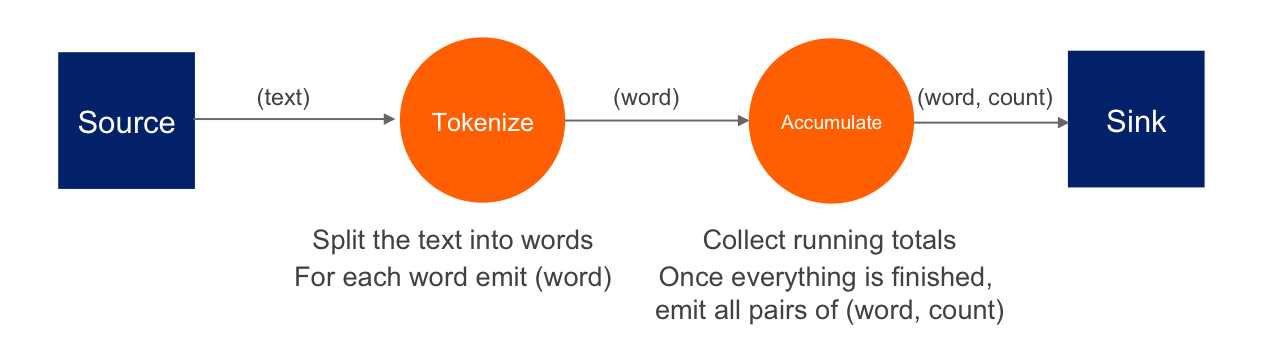The simplest, single-threaded code (shown above) deals with each item as it is produced: the outer loop reads the lines, the inner loop that runs for each line deals with the words on that line, and inside the inner loop we populate the result map with running counts.

However, just by modeling the computation as a DAG, we've split the work into isolated steps with clear data interfaces between them. We can perform the same computation by running a separate thread for each step. Roughly speaking, these are the snippets the threads would be executing:

``````// Source thread
for (String line : readLines()) {
emit(line);
}
``````
``````// Tokenizer thread
for (String line : receive()) {
for (String word : line.toLowerCase().split("\\W+")) {
if (!word.isEmpty()) {
emit(word);
}
}
}
``````
``````// Accumulator thread
Map<String, Long> counts = new HashMap<>();
for (String word : receive()) {
counts.merge(word, 1L, (count, one) -> count + one);
}
// finally, when done receiving:
for (Entry<String, Long> wordAndCount : counts.entrySet()) {
emit(wordAndCount);
}
``````

The source loop feeds the tokenizer loop over a concurrent queue, the tokenizer feeds the accumulator loop, and after the accumulator is done receiving, it emits its results to the sink. Diagrammatically it looks like this: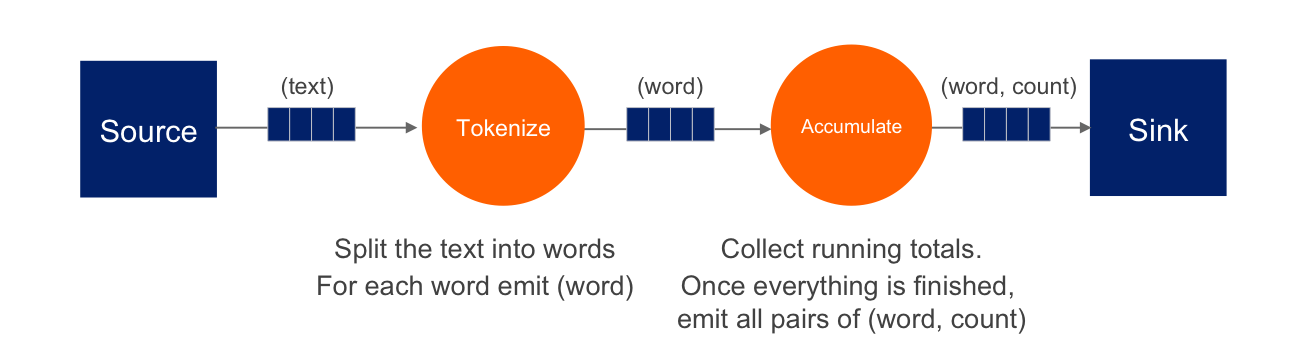This transformation brought us a pipelined architecture: while the tokenizer is busy with the regex work, the accumulator is updating the map using the data the tokenizer is done with; and the source and sink stages are pumping the data from/to the environment. Our design is now able to engage more than one CPU core and will complete that much sooner; however, we're still limited by the number of vertices. We'll be able utilize two or three cores regardless of how many are available. To move forward we must try to parallelize the work of each individual vertex.

Given that our input is an in-memory list of lines, the bottleneck occurs in the processing stages (tokenizing and accumulating). Let's first attack the tokenizing stage: it is a so-called "embarrassingly parallelizable" task because the processing of each line is completely self-contained. At this point we have to make a clear distinction between the notions of vertex and processor: there can be several processors doing the work of a single vertex. Let's add another tokenizing processor: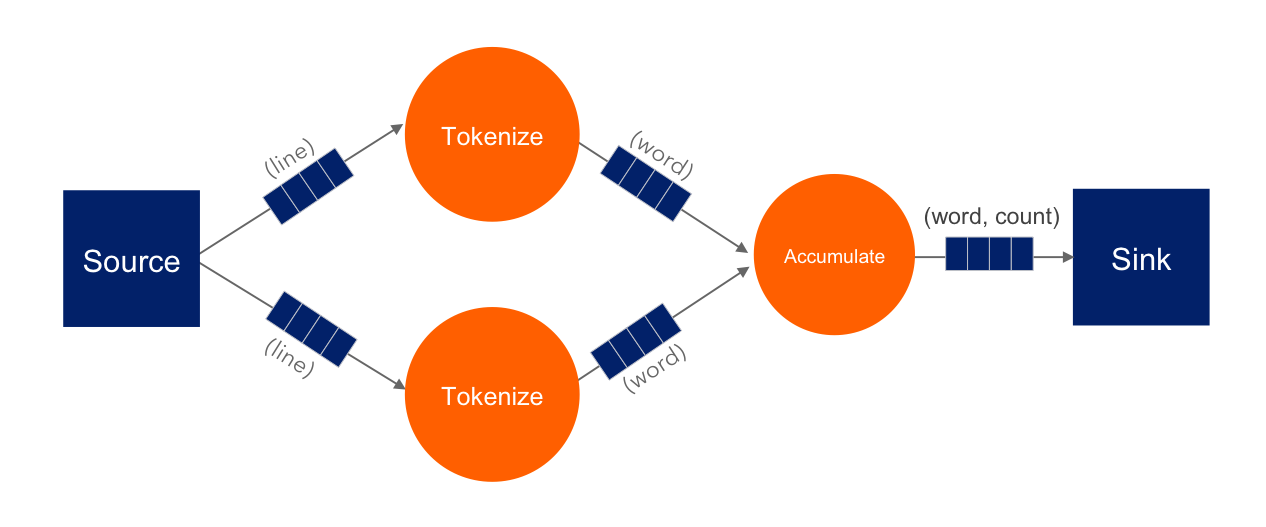The input processor can now use all the available tokenizers as a pool and submit to any one whose queue has some room.

The next step is parallelizing the accumulator vertex, but this is trickier: accumulators count word occurrences so using them as a pool will result in each processor observing almost all distinct words (entries taking space in its hashtable), but the counts will be partial and will need combining. The common strategy to reduce memory usage is to ensure that all occurrences of the same word go to the same processor. This is called "data partitioning" and in Jet we'll use a partitioned edge between the tokenizer and the accumulator: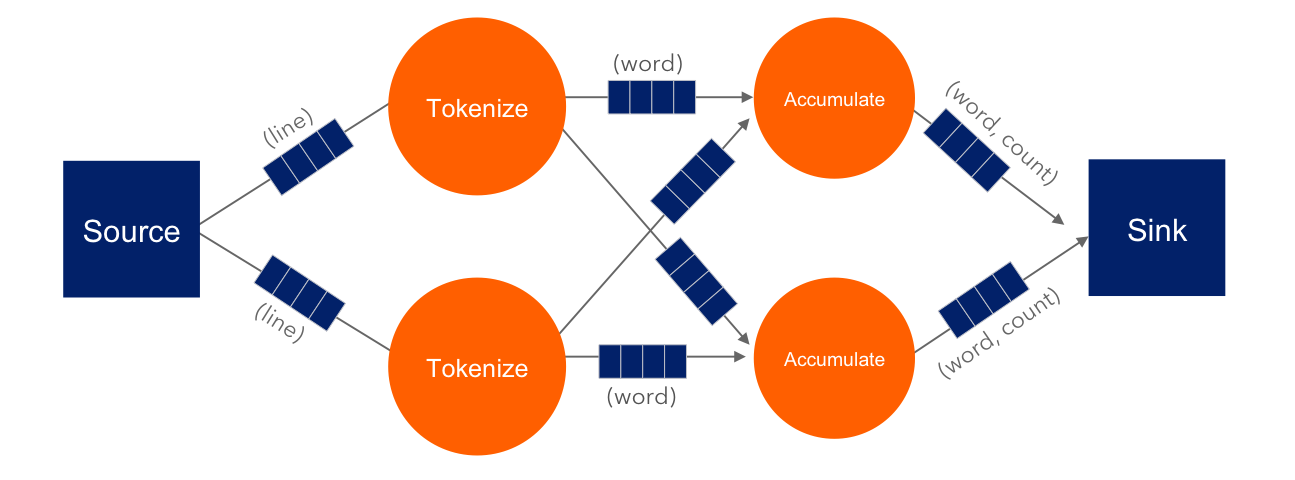As a word is emitted from the tokenizer, it goes through a "switchboard" stage where it's routed to the correct downstream processor. To determine where a word should be routed, we can calculate its hashcode and use the lowest bit to address either accumulator 0 or accumulator 1.

At this point we have a blueprint for a fully functional parallelized computation job which can max out all the CPU cores given enough instances of tokenizing and accumulating processors. The next challenge is making this work across machines.

For starters, our input can no longer be a simple in-memory list because that would mean each machine processes the same data. To exploit the cluster as a unified computation device, each cluster member must observe only a slice of the dataset. Given that a Jet instance is also a fully functional Hazelcast IMDG instance and a Jet cluster is also a Hazelcast IMDG cluster, the natural choice is to pre-load our data into an `IMap`, which will be automatically partitioned and distributed across the members. Now each Jet member can just read the slice of data that was stored locally on it.

When run in a cluster, Jet will instantiate a replica of the whole DAG on each member. On a two-member cluster there will be two source processors, four tokenizers, and so on. The trickiest part is the partitioned edge between tokenizer and accumulator: each accumulator is supposed to receive its own subset of words. That means that, for example, a word emitted from tokenizer 0 will have to travel across the network to reach accumulator 3, if that's the one that happens to own it. On average we can expect every other word to need network transport, causing both serious network traffic and serialization/deserialization CPU load.

There is a simple trick we can employ to avoid most of this traffic, closely related to what we pointed above as a source of problems when parallelizing locally: members of the cluster can be used as a pool, each doing its own partial word counts, and then send their results to a combining vertex. Note that this means sending only one item per distinct word. Here's the rough equivalent of the code the combining vertex executes:

``````// Combining vertex
Map<String, Long> combined = new HashMap<>();
for (Entry<String, Long> wordAndCount : receive()) {
combined.merge(wordAndCount.getKey(), wordAndCount.getValue(),
(accCount, newCount) -> accCount + newCount);
}
// finally, when done receiving:
for (Entry<String, Long> wordAndCount : combined.entrySet()) {
emit(wordAndCount);
}
``````

As noted above, such a scheme takes more memory due to more hashtable entries on each member, but it saves network traffic (an issue we didn't have within a member). Given that memory costs scale with the number of distinct keys (english words in our case), the memory cost is more-or-less constant regardless of how much book material we process. On the other hand, network traffic scales with the total data size so the more material we process, the more we save on network traffic.

Jet distinguishes between local and distributed edges, so we'll use a local partitioned edge for `tokenize`->`accumulate` and a distributed partitioned edge for `accumulate`->`combine`. With this move we've finalized our DAG design, which can be illustrated by the following diagram: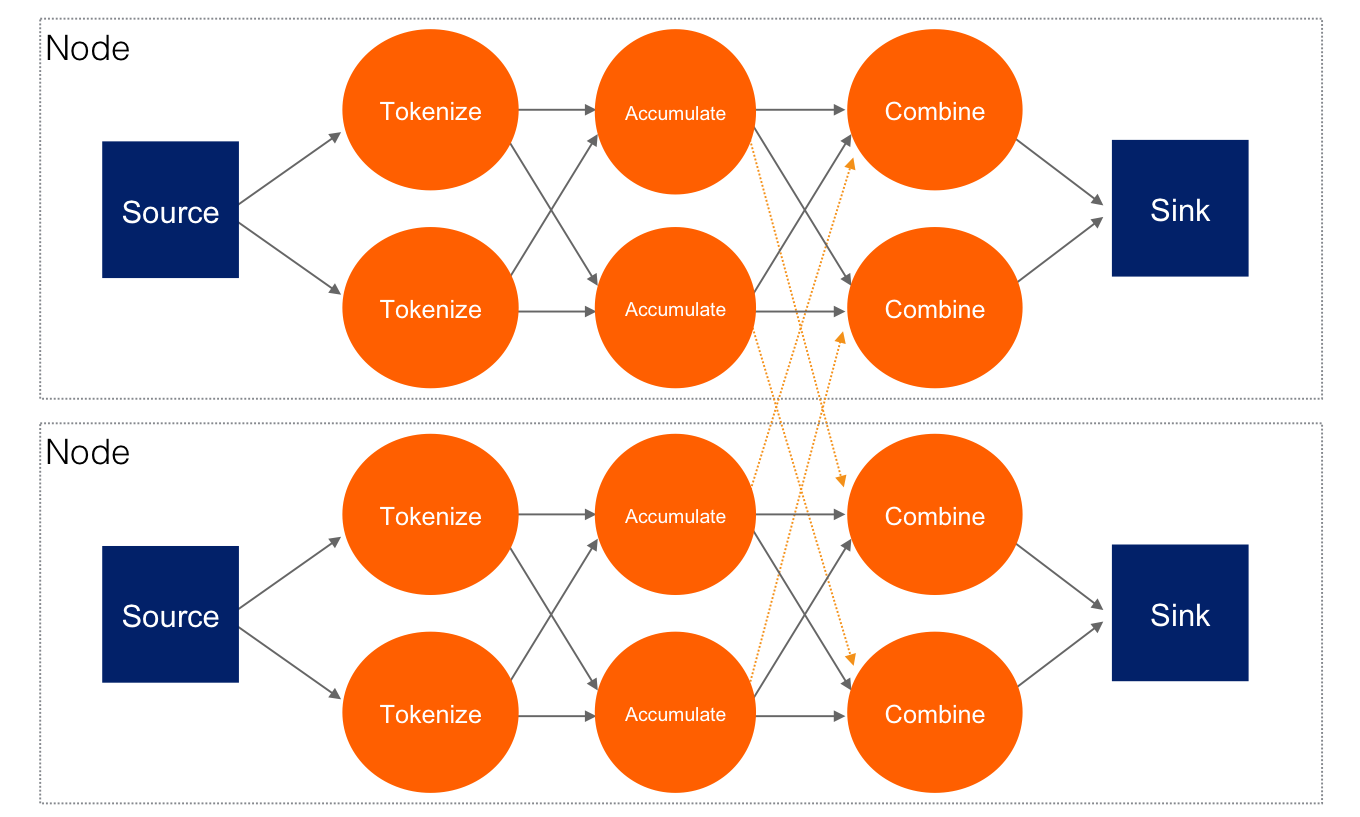## Implementing the DAG in Jet's Core API

Now that we've come up with a good DAG design, we can use Jet's Core API to implement it. We start by instantiating the DAG class and adding the source vertex:

``````DAG dag = new DAG();
``````

Note how we can build the DAG outside the context of any running Jet instances: it is a pure POJO.

The source vertex will read the lines from the `IMap` and emit items of type `Map.Entry<Integer, String>` to the next vertex. The key of the entry is the line number, and the value is the line itself. The built-in map-reading processor will do just what we want: on each member it will read only the data local to that member.

The next vertex is the tokenizer, which does a simple "flat-mapping" operation (transforms one input item into zero or more output items). The low-level support for such a processor is a part of Jet's library, we just need to provide the mapping function:

``````// (lineNum, line) -> words
Pattern delimiter = Pattern.compile("\\W+");
Vertex tokenize = dag.newVertex("tokenize",
Processors.flatMapP((Entry<Integer, String> e) ->
traverseArray(delimiter.split(e.getValue().toLowerCase()))
.filter(word -> !word.isEmpty()))
);
``````

This creates a processor that applies the given function to each incoming item, obtaining zero or more output items, and emits them. Specifically, our processor accepts items of type `Entry<Integer, String>`, splits the entry value into lowercase words, and emits all non-empty words. The function must return a `Traverser`, which is a functional interface used to traverse a sequence of non-null items. Its purpose is equivalent to the standard Java `Iterator`, but avoids the cumbersome two-method API. Since a lot of support for cooperative multithreading in Hazelcast Jet deals with sequence traversal, this abstraction simplifies many of its aspects.

The next vertex will do the actual word count. We can use the built-in `accumulateByKey` processor for this:

``````// word -> (word, count)
Vertex accumulate = dag.newVertex("accumulate",
Processors.accumulateByKeyP(wholeItem(), counting())
);
``````

This processor maintains a hashtable that maps each distinct key to its accumulated value. We specify `wholeItem()` as the key extractor function: our input item is just the word, which is also the grouping key. The second argument is the kind of aggregate operation we want to perform — counting. We're relying on Jet's out-of-the-box definitions here, but it's easy to define your own aggregate operations and key extractors. The processor emits nothing until it has received all the input, and at that point it emits the hashtable as a stream of `Entry<String, Long>`.

Next is the combining step which computes the grand totals from individual members' contributions. This is the code:

``````// (word, count) -> (word, count)
Vertex combine = dag.newVertex("combine",
Processors.combineByKeyP(counting())
);
``````

`combineByKey` is designed to be used downstream of `accumulateByKey`, which is why it doesn't need an explicit key extractor. The aggregate operation must be the same as on `accumulateByKey`.

The final vertex is the sink — we want to store the output in another `IMap`:

``````Vertex sink = dag.newVertex("sink", SinkProcessors.writeMapP("counts"));
``````

Now that we have all the vertices, we must connect them into a graph and specify the edge type as discussed in the previous section. Here's all the code at once:

``````dag.edge(between(source, tokenize))
.edge(between(tokenize, accumulate)
.partitioned(wholeItem(), Partitioner.HASH_CODE))
.edge(between(accumulate, combine)
.distributed()
.partitioned(entryKey()))
.edge(between(combine, sink));
``````

Let's take a closer look at some of the edges. First, source to tokenizer:

``````.edge(between(tokenize, accumulate)
.partitioned(wholeItem(), Partitioner.HASH_CODE))
``````

We chose a local partitioned edge. For each word, there will be a processor responsible for it on each member so that no items must travel across the network. In the `partitioned()` call we specify two things: the function that extracts the partitioning key (`wholeItem()` — same as the grouping key extractor), and the policy object that decides how to compute the partition ID from the key. Here we use the built-in `HASH_CODE`, which will derive the ID from `Object.hashCode()`. As long as the the definitions of `equals()/hashCode()` on the key object match our expected notion of key equality, this policy is always safe to use on a local edge.

Next, the edge from the accumulator to the combiner:

``````.edge(between(accumulate, combine)
.distributed()
.partitioned(entryKey()))
``````

It is distributed partitioned: for each word there is a single `combiner` processor in the whole cluster responsible for it and items will be sent over the network if needed. The partitioning key is again the word, but here it is the key part of the `Map.Entry<String, Long>`. We are using the default partitioning policy here (Hazelcast's own partitioning scheme). It is the slower-but-safe choice on a distributed edge. Detailed inspection shows that hashcode-based partitioning would be safe as well because all of `String`, `Long`, and `Map.Entry` have the hash function specified in their Javadoc.

You can acces a full, self-contained Java program with the above DAG code at the Hazelcast Jet code samples repository.